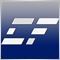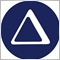Need help to understand PlotIndexSetInteger function6533

Hi,

Could anyone please help me understand what PlotIndexSetInteger() function does and its parameters?

Documentation section of this site doesn't explain it too well.

I'm looking at the code of MACD indicator... any input is greatly appreciated.

Thank you,Moderator
11158

BlindMist:

Hi,

Could anyone please help me understand what PlotIndexSetInteger() function does and its parameters?

Documentation section of this site doesn't explain it too well.

I'm looking at the code of MACD indicator... any input is greatly appreciated.

Thank you,

Hello Sergei,

did you take a look at the code base? I think there you can find good examples of how to use the MACD indicator.

Regarding the PlotIndexSetInteger function, it's not clear (at least to me) what exactly you need.

Please show us what you've done so far, so we can help you somehow.6533

Malacarne:

Hello Sergei,

did you take a look at the code base? I think there you can find good examples of how to use the MACD indicator.

Regarding the PlotIndexSetInteger function, it's not clear (at least to me) what exactly you need.

Please show us what you've done so far, so we can help you somehow.

Hi Rodrigo,

I'm attaching the following MACD code.

I don't really understand parameters of the following function:

PlotIndexSetInteger(1,PLOT_DRAW_BEGIN,InpSignalSMA-1);

For instance, the indicator has 4 buffers. Does the first parameter refer to the indicator's buffer number (i.e. '1')?

Removing this piece of code altogether doesn't seem to affect how indicator works.

//+------------------------------------------------------------------+
//|                                                         MACD.mq5 |
//|                        Copyright 2009, MetaQuotes Software Corp. |
//|                                              http://www.mql5.com |
//+------------------------------------------------------------------+
#property copyright   "2009, MetaQuotes Software Corp."
#property description "Moving Average Convergence/Divergence"
#include <MovingAverages.mqh>
//--- indicator settings
#property indicator_separate_window
#property indicator_buffers 4
#property indicator_plots   2
#property indicator_type1   DRAW_HISTOGRAM
#property indicator_type2   DRAW_LINE
#property indicator_color1  Silver
#property indicator_color2  Red
#property indicator_width1  2
#property indicator_width2  1
#property indicator_label1  "MACD"
#property indicator_label2  "Signal"
//--- input parameters
input int                InpFastEMA=12;               // Fast EMA period
input int                InpSlowEMA=26;               // Slow EMA period
input int                InpSignalSMA=9;              // Signal SMA period
input ENUM_APPLIED_PRICE InpAppliedPrice=PRICE_CLOSE; // Applied price
//--- indicator buffers
double                   ExtMacdBuffer[];
double                   ExtSignalBuffer[];
double                   ExtFastMaBuffer[];
double                   ExtSlowMaBuffer[];
//--- MA handles
int                      ExtFastMaHandle;
int                      ExtSlowMaHandle;
//+------------------------------------------------------------------+
//| Custom indicator initialization function                         |
//+------------------------------------------------------------------+
void OnInit()
{
//--- indicator buffers mapping
SetIndexBuffer(0,ExtMacdBuffer,INDICATOR_DATA);
SetIndexBuffer(1,ExtSignalBuffer,INDICATOR_DATA);
SetIndexBuffer(2,ExtFastMaBuffer,INDICATOR_CALCULATIONS);
SetIndexBuffer(3,ExtSlowMaBuffer,INDICATOR_CALCULATIONS);
//--- sets first bar from what index will be drawn
PlotIndexSetInteger(1,PLOT_DRAW_BEGIN,InpSignalSMA-1);
//--- name for Dindicator subwindow label
IndicatorSetString(INDICATOR_SHORTNAME,"MACD("+string(InpFastEMA)+","+string(InpSlowEMA)+","+string(InpSignalSMA)+")");
//--- get MA handles
ExtFastMaHandle=iMA(NULL,0,InpFastEMA,0,MODE_EMA,InpAppliedPrice);
ExtSlowMaHandle=iMA(NULL,0,InpSlowEMA,0,MODE_EMA,InpAppliedPrice);
//--- initialization done
}
//+------------------------------------------------------------------+
//| Moving Averages Convergence/Divergence                           |
//+------------------------------------------------------------------+
int OnCalculate(const int rates_total,const int prev_calculated,
const datetime &Time[],
const double &Open[],
const double &High[],
const double &Low[],
const double &Close[],
const long &TickVolume[],
const long &Volume[],
{
//--- check for data
if(rates_total<InpSignalSMA)
return(0);
//--- not all data may be calculated
int calculated=BarsCalculated(ExtFastMaHandle);
if(calculated<rates_total)
{
Print("Not all data of ExtFastMaHandle is calculated (",calculated,"bars ). Error",GetLastError());
return(0);
}
calculated=BarsCalculated(ExtSlowMaHandle);
if(calculated<rates_total)
{
Print("Not all data of ExtSlowMaHandle is calculated (",calculated,"bars ). Error",GetLastError());
return(0);
}
//--- we can copy not all data
int to_copy;
if(prev_calculated>rates_total || prev_calculated<0) to_copy=rates_total;
else
{
to_copy=rates_total-prev_calculated;
if(prev_calculated>0) to_copy++;
}
//--- get Fast EMA buffer
if(IsStopped()) return(0); //Checking for stop flag
if(CopyBuffer(ExtFastMaHandle,0,0,to_copy,ExtFastMaBuffer)<=0)
{
Print("Getting fast EMA is failed! Error",GetLastError());
return(0);
}
//--- get SlowSMA buffer
if(IsStopped()) return(0); //Checking for stop flag
if(CopyBuffer(ExtSlowMaHandle,0,0,to_copy,ExtSlowMaBuffer)<=0)
{
Print("Getting slow SMA is failed! Error",GetLastError());
return(0);
}
//---
int limit;
if(prev_calculated==0)
limit=0;
else limit=prev_calculated-1;
//--- calculate MACD
for(int i=limit;i<rates_total && !IsStopped();i++)
ExtMacdBuffer[i]=ExtFastMaBuffer[i]-ExtSlowMaBuffer[i];
//--- calculate Signal
SimpleMAOnBuffer(rates_total,prev_calculated,0,InpSignalSMA,ExtMacdBuffer,ExtSignalBuffer);
//--- OnCalculate done. Return new prev_calculated.
return(rates_total);
}39055

BlindMist:

Hi Rodrigo,

I'm attaching the following MACD code.

I don't really understand parameters of the following function:

For instance, the indicator has 4 buffers. Does the first parameter refer to the indicator's buffer number (i.e. '1')?

Removing this piece of code altogether doesn't seem to affect how indicator works.

There are 4 buffers, but only 2 plots (0= Histogram, 1= Line). So this command :

PlotIndexSetInteger(1,PLOT_DRAW_BEGIN,InpSignalSMA-1);

refer to plot 1, the line (Signal), which is a MA of the MACD (Main). DRAW_BEGIN results is that the line if only drawn after InpSignalMA candles, as you need some data before calculating a MA. It's a the start of the chart so you don't notice it.6533

angevoyageur:

There are 4 buffers, but only 2 plots (0= Histogram, 1= Line). So this command :

refer to plot 1, the line (Signal), which is a MA of the MACD (Main). DRAW_BEGIN results is that the line if only drawn after InpSignalMA candles, as you need some data before calculating a MA. It's a the start of the chart so you don't notice it.

Thanks!